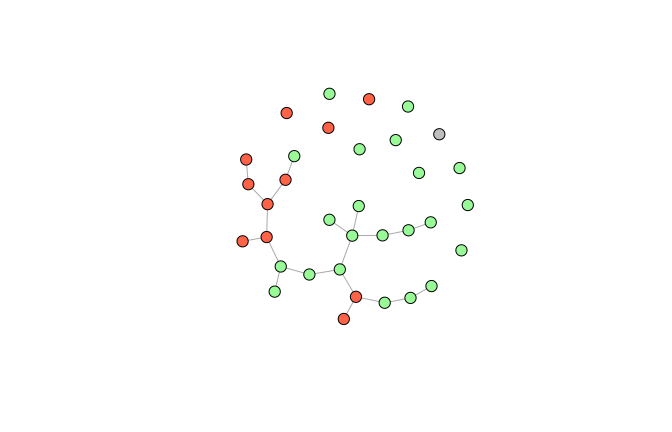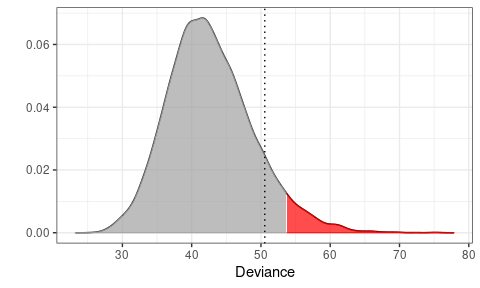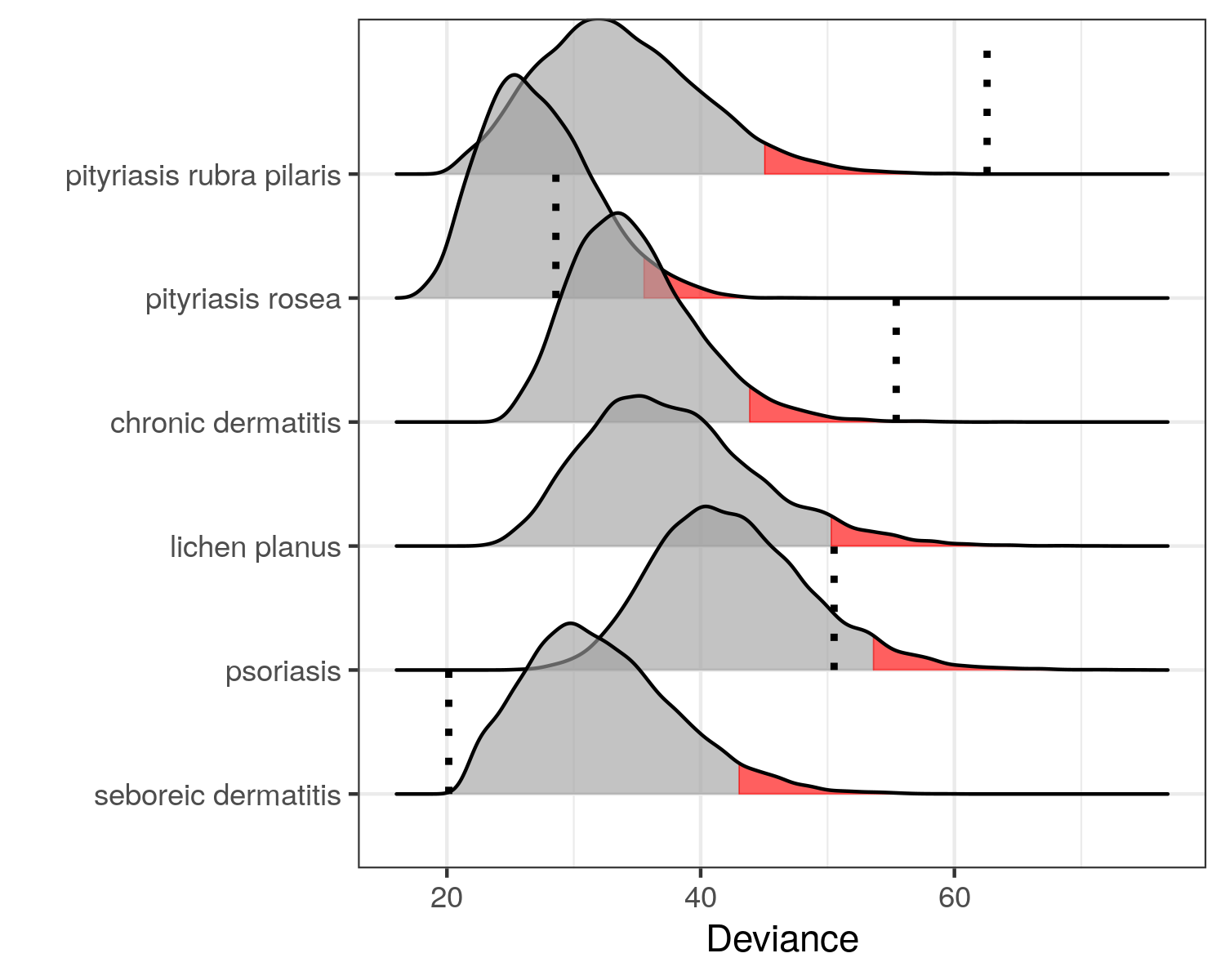# Detecting Skin Diseases

## Dermatitis

The UCI data set (Ilter and Altay Güvenir 1998) consist of

• $$366$$ patients ($$8$$ with missing values)
• $$12$$ clinical attributes (erythema, itching, scaling, etc.)
• $$21$$ histopathological attributes (decrease of melanin, spongiosis, etc.)

and a class variable with six different skin diseases

• psoriasis ($$111$$ patients)
• seborrheic dermatitis ($$60$$ patients)
• lichen planus ($$71$$ patients)
• pityriasis rosea ($$48$$ patients)
• chronic dermatitis ($$48$$ patients)
• pityriasis rubra pilaris ($$20$$ patients)

Many of the classical machine learning algorithms have been applied to the dataset (Liu et al. 2015). They all achieve a prediction accuracy above $$95\%$$ and some even above $$99\%$$. But…:

• The skin diseases share many common features
• We have exclusive classes but possibly not exhaustive
• What if a patient does not suffer from one of the six diseases
• Classification forces us to label a patient with one and only one disease

## Goal

Given a new patient $$y$$, we want to test the hypotheses

\begin{align*} H_1: & y \text{ has psoriasis} \\ H_2: & y \text{ has seborrheic dermatitis} \\ H_3: & y \text{ has lichen planus} \\ H_4: & y \text{ has pityriasis rosea} \\ H_5: & y \text{ has chronic dermatitis} \\ H_6: & y \text{ has pityriasis rubra pilaris} \end{align*}

Since all hypotheses are exclusive we do not correct for multiple hypothesis testing (but the user can do this by setting the significance level accordingly).

## Modeling Attributes of Psoriasis

We first show how to test $$H_1$$. First extract the psoriasis data:

library(dplyr)
library(molic)
y     <- unlist(derma[80, -35]) # a patient with seboreic dermatitis
psor  <- derma %>%
filter(ES == "psoriasis") %>%
dplyr::select(-ES)

Next, we fit the interaction graph for the psoriasis patients:

library(ess)
g <- fit_graph(psor, q = 0, trace = FALSE)

We can color the nodes corresponding to clinical attributes (red), histopathological attributes (green) and the age variable (gray):

vs   <- names(adj_lst(g))
vcol <- structure(vector("character", length(vs)), names = vs)
vcol[grepl("c", vs)] <- "tomato"  # clinical attributes
vcol[grepl("h", vs)] <- "#98FB98" # histopathological attributes
vcol["age"]          <- "gray"    # age variable
plot(g, vcol, vertex.size = 10, vertex.label = NA)The take home message here is, that we cannot assume independence between the attributes for the psoriasis patient as seen in the interaction graph - there are many associations.

## Outlier Model for Psoriasis Patients

set.seed(300718)
m <- fit_outlier(psor, g, y)
print(m)

--------------------------------
Simulations: 10000
Variables: 34
Observations: 112
Estimated mean: 42.74
Estimated variance: 37.73
--------------------------------
Critical value: 53.70377
Deviance: 50.55186
P-value: 0.1054
Alpha: 0.05
<novelty, outlier_model, list>
--------------------------------

Notice that that the number of observations is $$112$$ even though we have only observed $$111$$ psoriasis patients. This is because, under the hypothesis, $$H_1$$, the new observation $$y$$ has psoriasis. The other summary statistics is self explanatory.

## Plotting the Approximated Density of the Test Statistic

plot(m)The red area is the critical region (here 5%) and the dotted line is the observed test statistic (the deviance) of $$y$$. Since the dotted line is outside the critical region, we cannot reject that $$y$$ has psoriasis.

## Testing all Hypothesis Simultaneously

We can use the fit_multiple_models function to test all six hypothesis as follows.

set.seed(300718)
mm <- fit_multiple_models(derma, y, "ES", q = 0,trace = FALSE)
plot(mm)Thus, we cannot reject that $$y$$ has either psoriasis, seboreic dermatitis or pityriasis rosea. This is conservative compared to classification methods and hence a little safer. The medical expert should proceed the investigation from here.